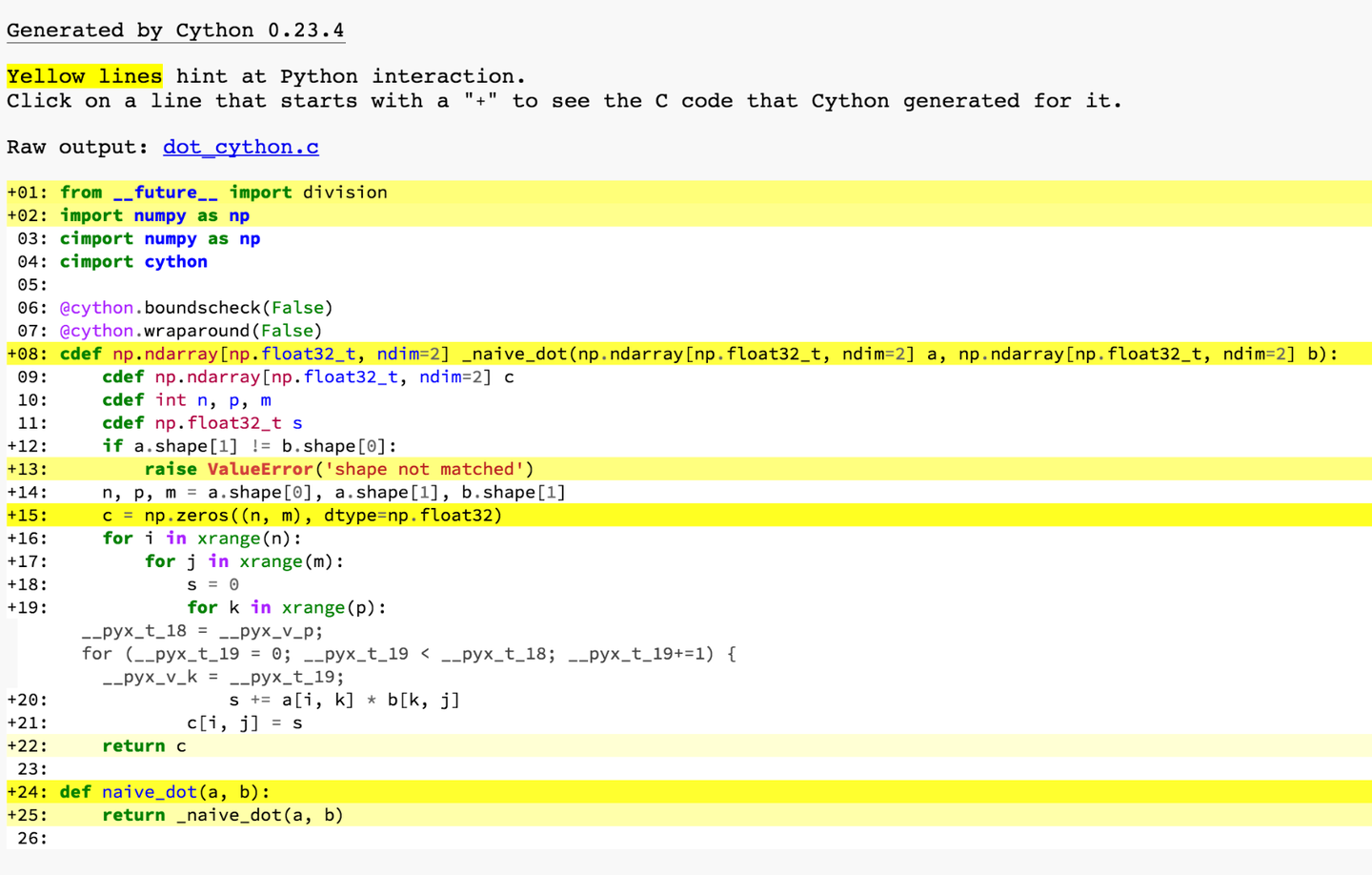​我一直非常喜欢 Python。当人们提到 Python 的时候，经常会说到下面两个优点：

1. 写起来方便
2. 容易调用 C/C++ 的库

• 用 Python 的语法混合编写 Python 和 C/C++ 代码，提升 Python 速度
• 调用 C/C++ 代码

## 例子：矩阵乘法

``````# dot_python.py
import numpy as np

def naive_dot(a, b):
if a.shape != b.shape:
raise ValueError('shape not matched')
n, p, m = a.shape, a.shape, b.shape
c = np.zeros((n, m), dtype=np.float32)
for i in xrange(n):
for j in xrange(m):
s = 0
for k in xrange(p):
s += a[i, k] * b[k, j]
c[i, j] = s
return c
``````

``````# dot_cython.pyx
import numpy as np
cimport numpy as np
cimport cython

@cython.boundscheck(False)
@cython.wraparound(False)
cdef np.ndarray[np.float32_t, ndim=2] _naive_dot(np.ndarray[np.float32_t, ndim=2] a, np.ndarray[np.float32_t, ndim=2] b):
cdef np.ndarray[np.float32_t, ndim=2] c
cdef int n, p, m
cdef np.float32_t s
if a.shape != b.shape:
raise ValueError('shape not matched')
n, p, m = a.shape, a.shape, b.shape
c = np.zeros((n, m), dtype=np.float32)
for i in xrange(n):
for j in xrange(m):
s = 0
for k in xrange(p):
s += a[i, k] * b[k, j]
c[i, j] = s
return c

def naive_dot(a, b):
return _naive_dot(a, b)
``````

• Cython 程序的扩展名是 .pyx
• cimport 是 Cython 中用来引入 .pxd 文件的命令。有关 .pxd 文件，可以简单理解成 C/C++ 中用来写声明的头文件，更具体的我会在后面写到。这里引入的两个是 Cython 预置的。
• @cython.boundscheck(False) 和 @cython.wraparound(False) 两个修饰符用来关闭 Cython 的边界检查
• Cython 的函数使用 cdef 定义，并且他可以给所有参数以及返回值指定类型。比方说，我们可以这么编写整数 min 函数：

``````  cdef int my_min(int x, int y):
return x if x <= y else y
``````

这里 np.ndarray[np.float32_t, ndim=2] 就是一个类型名就像 int 一样，只是它比较长而且信息量比较大而已。它的意思是，这是个类型为 np.float32_t 的2维 np.ndarray。

• 在函数体内部，我们一样可以使用 cdef typename varname 这样的语法来声明变量
• 在 Python 程序中，是看不到 cdef 的函数的，所以我们这里 def naive_dot(a, b) 来调用 cdef 过的 _naive_dot 函数。

1. Cython 编译器把 Cython 代码编译成调用了 Python 源码的 C/C++ 代码
2. 把生成的代码编译成动态链接库
3. Python 解释器载入动态链接库

``````# setup.py
from distutils.core import setup, Extension
from Cython.Build import cythonize
import numpy
setup(ext_modules = cythonize(Extension(
'dot_cython',
sources=['dot_cython.pyx'],
language='c',
include_dirs=[numpy.get_include()],
library_dirs=[],
libraries=[],
extra_compile_args=[],
)))
``````

• 'dot_cython' 是我们要生成的动态链接库的名字
• sources 里面可以包含 .pyx 文件，以及后面如果我们要调用 C/C++ 程序的话，还可以往里面加 .c / .cpp 文件
• language 其实默认就是 c，如果要用 C++，就改成 c++ 就好了
• include_dirs 这个就是传给 gcc 的 -I 参数
• library_dirs 这个就是传给 gcc 的 -L 参数
• libraries 这个就是传给 gcc 的 -l 参数
• extra_compile_args 就是传给 gcc 的额外的编译参数，比方说你可以传一个 -std=c++11
• 如果你从来没见过上面几个 gcc 参数，说明你暂时还没这些需求，等你遇到了你就懂了

``````python setup.py build_ext --inplace
``````

``````\$ ipython                                                                                                   15:07:43
Python 2.7.12 (default, Oct 11 2016, 05:20:59)

IPython 4.0.1 -- An enhanced Interactive Python.
?         -> Introduction and overview of IPython's features.
%quickref -> Quick reference.
help      -> Python's own help system.
object?   -> Details about 'object', use 'object??' for extra details.

In : import numpy as np
In : import dot_python
In : import dot_cython
In : a = np.random.randn(100, 200).astype(np.float32)
In : b = np.random.randn(200, 50).astype(np.float32)

In : %timeit -n 100 -r 3 dot_python.naive_dot(a, b)
100 loops, best of 3: 560 ms per loop

In : %timeit -n 100 -r 3 dot_cython.naive_dot(a, b)
100 loops, best of 3: 982 µs per loop

In : %timeit -n 100 -r 3 np.dot(a, b)
100 loops, best of 3: 49.2 µs per loop
``````

``````// dot.cpp
#include <ctime>
#include <cstdlib>
#include <chrono>
#include <iostream>

class Matrix {
float *data;
public:
size_t n, m;
Matrix(size_t r, size_t c): data(new float[r*c]), n(r), m(c) {}
~Matrix() { delete[] data; }
float& operator() (size_t x, size_t y) { return data[x*m+y]; }
float operator() (size_t x, size_t y) const { return data[x*m+y]; }
};

float dot(const Matrix &a, const Matrix& b) {
Matrix c(a.n, b.m);
for (size_t i = 0; i < a.n; ++i)
for (size_t j = 0; j < b.m; ++j) {
float s = 0;
for (size_t k = 0; k < a.m; ++k)
s += a(i, k) * b(k, j);
c(i, j) = s;
}
return c(0, 0); // to comfort -O2 optimization
}

void fill_rand(Matrix &a) {
for (size_t i = 0; i < a.n; ++i)
for (size_t j = 0; j < a.m; ++j)
a(i, j) = rand() / static_cast<float>(RAND_MAX) * 2 - 1;
}

int main() {
srand((unsigned)time(NULL));
const int n = 100, p = 200, m = 50, T = 100;
Matrix a(n, p), b(p, m);
fill_rand(a);
fill_rand(b);
auto st = std::chrono::system_clock::now();
float s = 0;
for (int i = 0; i < T; ++i) {
s += dot(a, b);
}
auto ed = std::chrono::system_clock::now();
std::chrono::duration<double> diff = ed-st;
std::cerr << s << std::endl;
std::cout << T << " loops. average " << diff.count() * 1e6 / T << "us" << std::endl;
}
``````
``````\$ g++ -O2 -std=c++11 -o dot dot.cpp
\$ ./dot 2>/dev/null
100 loops. average 1112.11us
``````

## Cython 加速 Python 代码的关键

``````\$ python setup.py build_ext --inplace
\$ ipython
In : import numpy as np
In : import dot_python
In : import dot_cython
In : a = np.random.randn(100, 200).astype(np.float32)
In : b = np.random.randn(200, 50).astype(np.float32)

In : %timeit -n 100 -r 3 dot_cython.naive_dot(a, b)
100 loops, best of 3: 416 ms per loop

In : %timeit -n 100 -r 3 dot_python.naive_dot(a, b)
100 loops, best of 3: 537 ms per loop
``````

## 分析 Cython 程序

``````cython -a dot_cython.pyx
``````

## 作为胶水

Python 是很好的胶水语言，但是前提是库本身要使用 Python API 来和 Python 交互。有了 Cython 之后，我们可以照常编写 C/C++ 程序，或者是直接拿来一份已有的 C/C++ 源码，然后用 Cython 简单包装一下就可以使用了。

• 函数签名基本上可以原样从 C/C++ 复制到 Cython 中
• C 中的 _Bool 类型和 C++ 中的 bool 类型在 Cython 中都用 bint 取代（因为 Python 没有布尔类型）
• struct / enum / union 是支持的
• const 限定和引用都是支持的
• 命名空间是支持的
• C++ 类是支持的
• 部分操作符重载是支持的，部分操作符需要改名
• 内嵌类是支持的
• 模板是支持的
• 异常是支持的
• 构造函数、析构函数是支持的
• 静态成员是支持的
• libc / libcpp / STL 是支持的
• 声明写在 .pxd 中可以在 .pyx 中 cimport 进来
• 你可能需要注意 Python 字符串到各式各样的 C/C++ 字符串的转换

​首发于博客 Python 多核并行计算